Next: 12.3 Maxima and Minima Up: 12. Extreme Values of Previous: 12.1 Continuity   Index

# 12.2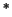A Nowhere Differentiable Continuous Function.

We will now give an example of a function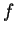that is continuous at every point of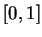and differentiable at no point of. The first published example of such a function appeared in 1874 and was due to Karl Weierstrass(1815-1897) [29, page 976]. The example described below is due to Helga von Koch (1870-1924), and is a slightly modified version of Koch's snowflake. From the discussion in section 2.6, it is not really clear what we would mean by the perimeter of a snowflake, but it is pretty clear that whatever the perimeter might be, it is not the graph of a function. However, a slight modification of Koch's construction yields an everywhere continuous but nowhere differentiable function.

We will construct a sequence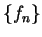of functions on. The graph of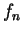will be a polygonal line with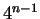segments. We set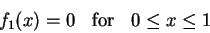so that the graph of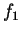is the line segment from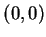to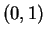.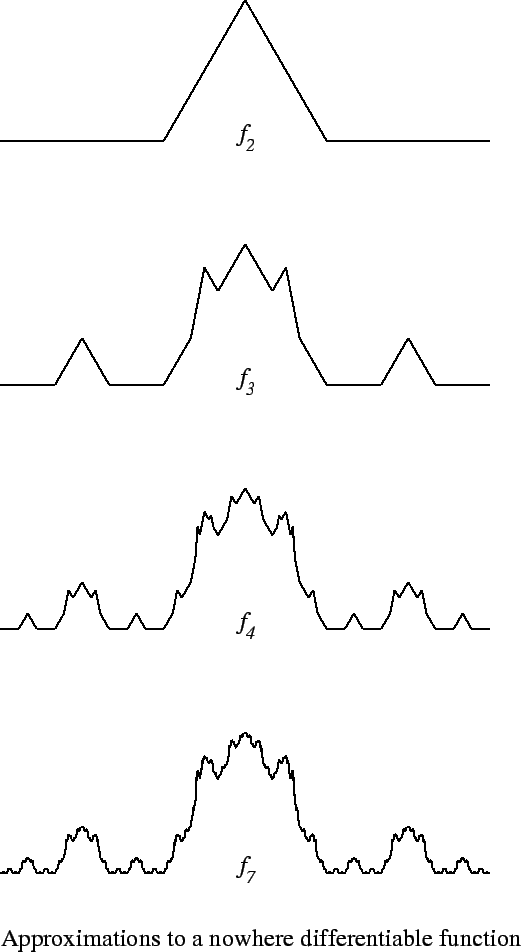In general the graph of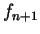is obtained from the graph ofby replacing each segment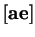in the graph ofby four segments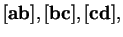and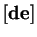constructed according to the following three rules: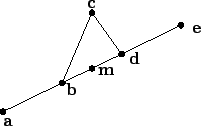i)
The points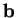and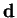trisect the segment.
ii)
The pointlies above the midpoint m of.
iii)
distance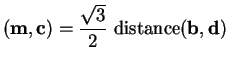.
The graphs of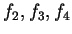and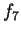are shown above . It can be shown that for each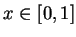the sequence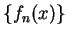converges. Defineonby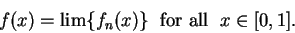It turns out thatis continuous onand differentiable nowhere on. A proof of this can be found in [31, page 168].

The functionprovides us with an example of a continuous function that is not piecewise monotonic over any interval.Next: 12.3 Maxima and Minima Up: 12. Extreme Values of Previous: 12.1 Continuity   Index
Ray Mayer 2007-09-07